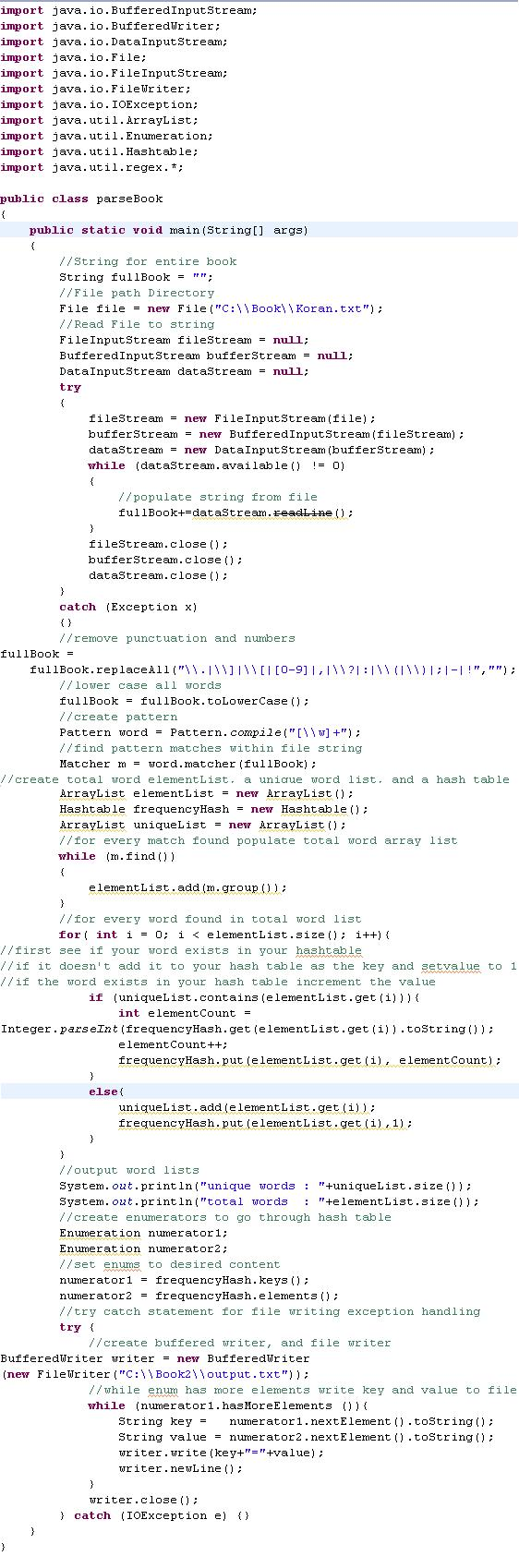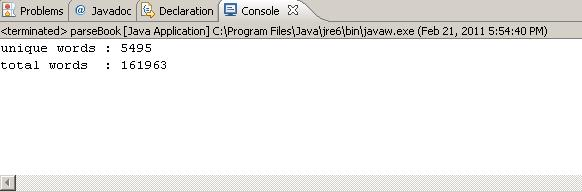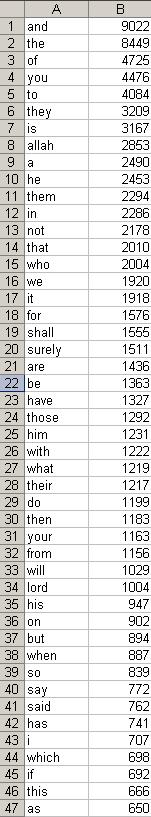# JAVA: How to parse every word in a book and output the frequency of repeating words: The Holy Koran

The book being parsed in this example is the Holy Koran.  (English Translation) Koran.txtColumn A is the word, Column B is the frequencymicrosoft excel format : korFreq.xls

xml format                     : korFreqxml.txt

full code

[java]
import java.io.BufferedInputStream;
import java.io.BufferedWriter;
import java.io.DataInputStream;
import java.io.File;
import java.io.FileInputStream;
import java.io.FileWriter;
import java.io.IOException;
import java.util.ArrayList;
import java.util.Enumeration;
import java.util.Hashtable;
import java.util.regex.*;

public class parseBook
{
public static void main(String[] args)
{
//String for entire book
String fullBook = “”;
//File path Directory
File file = new File(“C:\\Book\\Koran.txt”);
FileInputStream fileStream = null;
BufferedInputStream bufferStream = null;
DataInputStream dataStream = null;
try
{
fileStream = new FileInputStream(file);
bufferStream = new BufferedInputStream(fileStream);
dataStream = new DataInputStream(bufferStream);
while (dataStream.available() != 0)
{
//populate string from file
}
fileStream.close();
bufferStream.close();
dataStream.close();
}
catch (Exception x)
{}
//remove punctuation and numbers
fullBook =
fullBook.replaceAll(“\\.|\\]|\\[|[0-9]|,|\\?|:|\\(|\\)|;|-|!”,””);
//lower case all words
fullBook = fullBook.toLowerCase();
//create pattern
Pattern word = Pattern.compile(“[\\w]+”);
//find pattern matches within file string
Matcher m = word.matcher(fullBook);
//create total word elementList, a unique word list, and a hash table
ArrayList elementList = new ArrayList();
Hashtable frequencyHash = new Hashtable();
ArrayList uniqueList = new ArrayList();
//for every match found populate total word array list
while (m.find())
{
}
//for every word found in total word list
for( int i = 0; i < elementList.size(); i++){
//if it doesn’t add it to your hash table as the key and setvalue to 1
//if the word exists in your hash table increment the value
if (uniqueList.contains(elementList.get(i))){
int elementCount =
Integer.parseInt(frequencyHash.get(elementList.get(i)).toString());
elementCount++;
frequencyHash.put(elementList.get(i), elementCount);
}
else{
frequencyHash.put(elementList.get(i),1);
}
}
//output word lists
System.out.println(“unique words : “+uniqueList.size());
System.out.println(“total words : “+elementList.size());
//create enumerators to go through hash table
Enumeration numerator1;
Enumeration numerator2;
//set enums to desired content
numerator1 = frequencyHash.keys();
numerator2 = frequencyHash.elements();
//try catch statement for file writing exception handling
try {
//create buffered writer, and file writer
BufferedWriter writer = new BufferedWriter
(new FileWriter(“C:\\Book2\\output.txt”));
//while enum has more elements write key and value to file
while (numerator1.hasMoreElements ()){
String key = numerator1.nextElement().toString();
String value = numerator2.nextElement().toString();
writer.write(key+”=”+value);
writer.newLine();
}
writer.close();
} catch (IOException e) {}
}
}
[/java]

1. Dhivya Mj